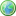Accessibility options:

# Simple linear equations resources

Show me all resources applicable to

### Test Yourself (5)Diagnostic Test - Linear equations
Diagnostic test for linear equations. This resource is released under a Creative Commons license Attribution-Non-Commercial-No Derivative Works and the copyright is held by Skillbank Solutions Ltd.Exercise - Linear equations
Online exercise on linear equations. This resource is released under a Creative Commons license Attribution-Non-Commercial-No Derivative Works and the copyright is held by Skillbank Solutions Ltd.Maths EG
Computer-aided assessment of maths, stats and numeracy from GCSE to undergraduate level 2. These resources have been made available under a Creative Common licence by Martin Greenhow and Abdulrahman Kamavi, Brunel University.Solving simple linear equations - Numbas
2 equations, both linear (the second needs a small amount of algebra to reduce to a linear equation). Numbas resources have been made available under a Creative Commons licence by the School of Mathematics & Statistics at Newcastle University.Solving simple linear equations - Numbas
2 equations, both linear (the second needs a small amount of algebra to reduce to a linear equation). Numbas resources have been made available under a Creative Commons licence by Bill Foster and Christian Perfect, School of Mathematics & Statistics at Newcastle University.

Website design by Pink Mayhem, Leicester